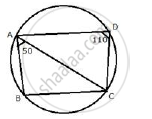Share

Abcd is a Cyclic Quadrilateral in Which Bc is Parallel to Ad, Angle Adc = 110° and Angle Bac = 50°. Find Angle Dac and Angle Dca. - ICSE Class 10 - Mathematics

Question

ABCD is a cyclic quadrilateral in which BC is parallel to AD, angle ADC = 110° and angle BAC = 50°. Find angle DAC and angle DCA.

SolutionABCD is a cyclic quadrilateral in which AD||BC
∠ADC =  110°  , ∠BAC = 50°
∠B + ∠D  = 180°
(Sum of opposite angles of a quadrilateral)
⇒ ∠B  + 110° = 180°
⇒ ∠B  = 70°
Now in ΔABC,
∠BAC + ∠ABC + ∠ACB  = 180°
⇒ 50° + 70° + ∠ACB  = 180°
⇒ ∠ACB  = 180° - 120° = 60°
∵ AD ll BC
∴ ∠DAC= ∠ACB = 60° (alternate angles)
∠DAC + ∠ADC +∠DCA = 180°
⇒ 60° + 110°  +∠DCA = 180°
⇒ ∠DCA = 180°  - 170° = 10°